• 对于常见的多布局，我们可以设置不同的type，例如 getItemViewType方法： if (mDatas.get(position).getType() ==1) { return 1; } else if (mDatas.get(position).getType() ==2) { return 2; } ...
对于常见的多布局，我们可以设置不同的type，例如 getItemViewType方法：


if (mDatas.get(position).getType() ==1) {

return   1;

} else if (mDatas.get(position).getType() ==2) {

return    2;

}


onCreateViewHolder方法

if (viewType == 1) {

/*显示服务的item项*/ view = LayoutInflater.from(mContext).inflate(R.layout.a,  parent ,   false);

return new AHolder(view);


} else if (viewType == 2) {

/*显示服务title项*/ view =     LayoutInflater.from(mContext).inflate(R.layout.b, parent, false);


return new BHolder(view);

onBindViewHolder方法同理

if (getItemViewType(position) == 1) {

((AHolder) holder).aTextview.setText("a");


}else{

}(( BHolder ) holder).aTextview.setText(“b”);

对于如果不同的viewType加载的list类型都不同，例如A类型需要的是Adata,类型B需要的是Bdata，一个list无法传递过来，我们需要把Adata和Bdata都继承Cdata，然后在Cdata中设置一个Type，添加不同数据类型时候设置不同type，传入判断当前type是A类型的数据还是B类型数据，强转不同数据类型加载数据
展开全文• 在进行测试的过程中，我们的样品...本文的内容一共分为文件打开，数据合并，数据保存三部分 1 文件打开： **#打开文件 def open(file_csv): df=pd.read_csv(filepath_or_buffer='c:\\Users\\dell\\Desktop\\test.csv',
在进行测试的过程中，我们的样品数量有很多，但是不同的样品测试完就形成了一个独立的文件，不同样品会形成不同的文件，因此，有时在进行数据处理之前，需要将这些数据列表进行合并统一分析，本文上传我的方法：
本文的内容一共分为文件打开，数据合并，数据保存三部分
1 文件打开：
**#打开文件
def open(file_csv):
encoding='ANSI',
skiprows=(11))
return df

为了使程序的的可读性变强，我们选择将功能打包成函数，每个函数只完成一个操作，以上代码的含义是：
定义一个open（file_csv）的函数：
encoding="ANSI"这是解码方式，也可以是UTF-8，这要根据具体的文件解码方式来选择；
skiprows=（11）是我选择跳过最开始的10行，因为他们不是我所需要的数据，并且会影响我需要数据的列名排列，因此这里选择跳过他们，这里需要注意：
skiprows=（11）是跳过最开始的0-11行，
skiprows=[0,2,5]是跳过0，2，5共分立的3行；
然后返回表格df
2 数据合并：
#合并数据
def contact(df1,df2):
df=pd.concat([df1,df2],ignore_index=True)
return df

定义一个contact（）的函数，用来连接df1和df2这两个表格形成一个新的表格，这两个表格拥有相同的索引；
所使用的是pd.concat()函数，这里要特别注意是concat不是contact噢，写错的话会报属性错误：
AttributeError: module 'pandas' has no attribute 'contcat'

[df1,df2]参数包括需要连接的数据对象，可以是列表或者字典，用[ ]括起来；
axis参数表示连接轴的方向，默认是0，沿着 行方向；
join参数表示连接方式，默认是"outer"是两个数据的并集；
ignore_index=True:意思是不沿着连接轴保留索引而产生一段新的索引
效果如下图所示：
df1：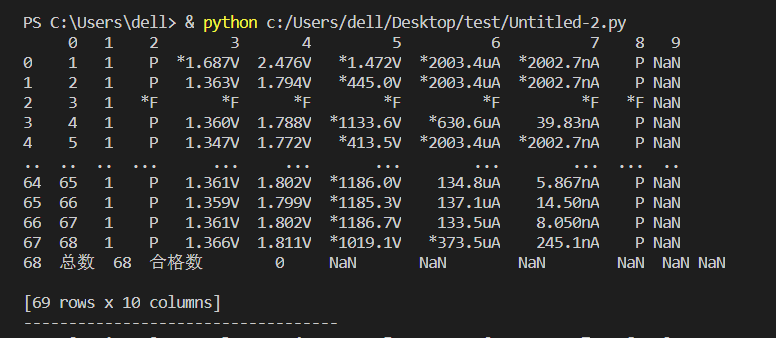df2：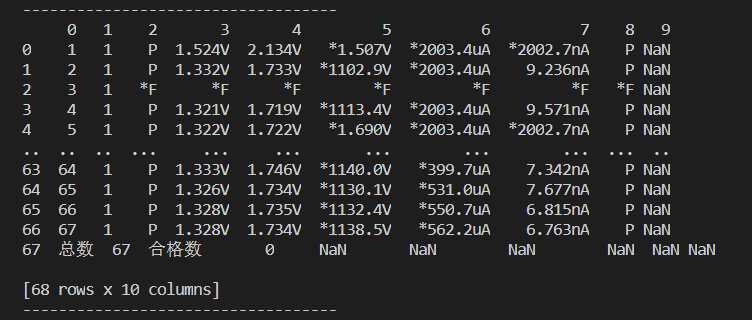contact（df1，df2）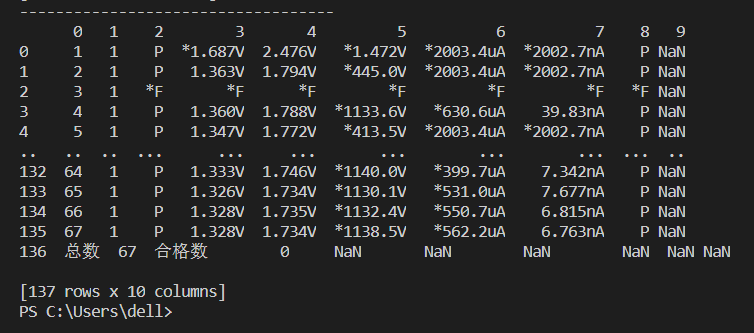代码样子：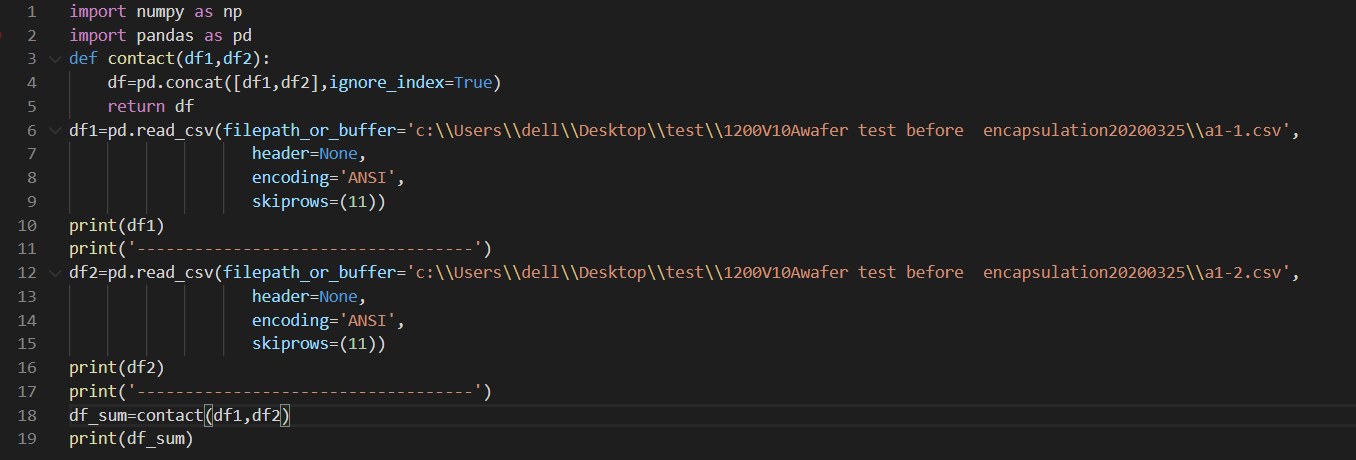3 数据保存
#数据保存
df_sum.to_csv(path_or_buf='c:\\Users\\dell\\Desktop\\test.csv',index=False)

使用的是保存为csv文件的函数，path_or_buf参数说明保存的地址，index=False使得索引行不在文件中占单独的一行，这样当我们调用该文件进行绘图时就不会出现可恶的unnamed：0行出现干扰
无干扰：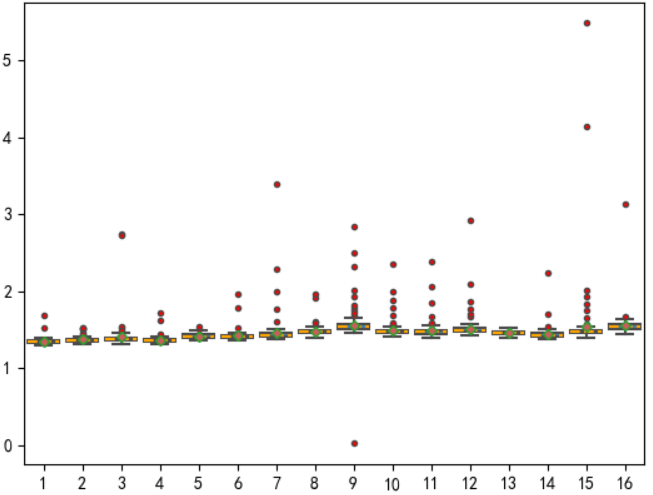有干扰：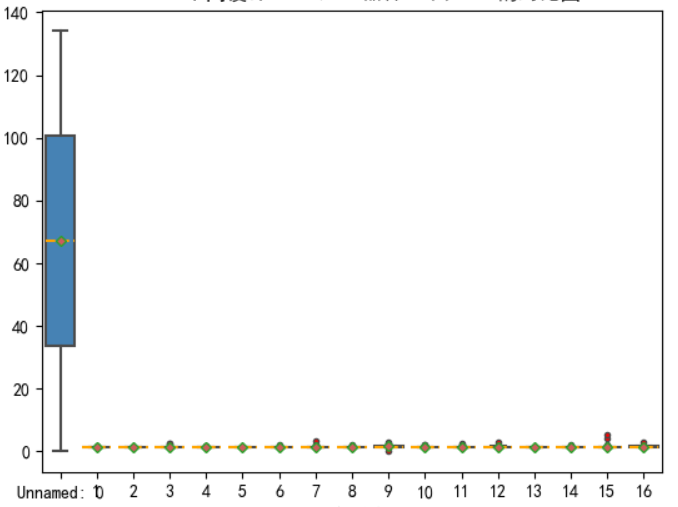由上图对比，可以发现这种unnamed：0列非常影响图像表达效果，要引起注意**


展开全文python
• ## pandas之数据合并

千次阅读 2018-06-23 13:38:07
今天要说的数据合并其实和数据表之间的连接有很多相似之处。由于 pandas 库在数据分析中比较方便而且用者较多，我们就说pandas中的数据合并方式。 pandas 中数据合并常用的方法有三种：pandas.merge(), pandas....
       在数据处理中，不免会遇到数据之间的合并。学过关系数据库的童鞋应该都清楚数据表之间的连接。今天要说的数据合并其实和数据表之间的连接有很多相似之处。由于 pandas 库在数据分析中比较方便而且用者较多，我们就说pandas中的数据合并方式。     pandas 中数据合并常用的方法有三种：pandas.merge(), pandas.concat(), 以及实例方法 combine_first()1）pandas.merge()merge函数的参数：left, right参与合并的左、右侧Dataframehowinner、outer、left、right之一，默认是 inneron指定用于连接的列名。如果未指定，则会自动选取要合并数据中相同的列名left_on, right_on左、右侧Dataframe用于连接键的列left_index, right_index将左 \ 右侧的行索引用作连接键sort根据连接键对合并后的数据进行排序，默认True。在处理大数据集的时候，禁用此功能能会有很好的性能提升suffixes字符串元组，用于追加到重复列名的末尾，默认('_x', '_y')copy设置为False，可以在某些特殊情况下 避免将数据复制到结果数据结构中。默认总是复制。看几个简单的例子：In : df1 = pd.DataFrame({'k':['s','s','w','x','x','n','f','c'],'data1':range(8)})
In : df2 = pd.DataFrame({'k':['w','w','s','s','x','f'],'data2':range(6)})
In : pd.merge(df1,df2)  # 未指定合并的列，默认选取两者重复的列 k， 也可以指定 pd.merge(df1,df2，on='k')
Out:
data1  k  data2
0      0  s      2
1      0  s      3
2      1  s      2
3      1  s      3
4      2  w      0
5      2  w      1
6      3  x      4
7      4  x      4
8      6  f      5从上面的结果中会发现有些行消失了，这是因为默认使用的是 inner 连接方式，结果做的交集。可以指定其他的连接方式(参见上面的 how 参数值)。例如：In : pd.merge(df1,df2,how='outer')
Out:
data1  k  data2
0       0  s    2.0
1       0  s    3.0
2       1  s    2.0
3       1  s    3.0
4       2  w    0.0
5       2  w    1.0
6       3  x    4.0
7       4  x    4.0
8       5  n    NaN
9       6  f    5.0
10      7  c    NaN合并的列名不同时，手动指定要合并的列：In : df2 = pd.DataFrame({'k_1':['w','w','s','s','x','f'] ,'data2':range(6)})
In : pd.merge(df1,df2,left_on='k',right_on='k_1',how='outer')
Out:
data1  k  data2  k_1
0       0  s    2.0    s
1       0  s    3.0    s
2       1  s    2.0    s
3       1  s    3.0    s
4       2  w    0.0    w
5       2  w    1.0    w
6       3  x    4.0    x
7       4  x    4.0    x
8       5  n    NaN  NaN
9       6  f    5.0    f
10      7  c    NaN  NaN根据多个键进行合并，只需要在 on 关键字传入一个列名组成的列表即可：In : left = pd.DataFrame({'key1': ['foo', 'foo', 'bar'],
...: 'key2': ['one', 'two', 'one'],'val': [1, 2, 3]})
In : right = pd.DataFrame({'key1': ['foo', 'foo','bar','bar'],
...: 'key2': ['one', 'one', 'one','two'],'val': [4,5,6,7]})

In : pd.merge(left, right, on=['key1', 'key2'], how='outer')
Out:
key1 key2  val_x  val_y
0  foo  one   1.0   4.0
1  foo  one   1.0   5.0
2  foo  two   2.0   NaN
3  bar  one   3.0   6.0
4  bar  two   NaN   7.0从上面的例子中还可以看到，当合并的数据有相同的列时，结果会默认在后面添加_x, _y来区分。也可以手动指定：In : pd.merge(left, right, on='key1', how='outer',suffixes=('_left','_right'))
Out:
key1 key2_left  val_left key2_right  val_right
0  foo       one         1        one          4
1  foo       one         1        one          5
2  foo       two         2        one          4
3  foo       two         2        one          5
4  bar       one         3        one          6
5  bar       one         3        two          7索引作为连接键的合并有的时候我们要合并的连接键在索引中，这种情况我们就要通过 left_index = True  \ right_index = True 来明确索引作为连接键。
In : df3 = pd.DataFrame({'data3':[5,2,0]},index=list('sxn'))
In : df3
Out:
data3
s      5
x      2
n      0
In : pd.merge(df1,df3,left_on='k',right_index=True,how='outer')
Out:
data1  k  data3
0      0  s    5.0
1      1  s    5.0
2      2  w    NaN
3      3  x    2.0
4      4  x    2.0
5      5  n    0.0
6      6  f    NaN
7      7  c    NaN合并层次化索引的数据，必须以列表的形式指明用作合并的列：In :  lefth = pd.DataFrame({'key1': ['sxn', 'sxn', 'sxn', 'wfc', 'wfc'],
'key2': [2000, 2001, 2002, 2001, 2002],
...: 'data': np.arange(5.)})
In : righth = pd.DataFrame(np.arange(12).reshape((6, 2)) ,
...: index=[['wfc', 'wfc', 'sxn', 'sxn', 'sxn', 'snx'],
...: [2001, 2000, 2000, 2000, 2001, 2002]],
...: columns=['event1', 'event2'])
In : pd.merge(lefth, righth, left_on=['key1', 'key2'],right_index=True, how='outer')
Out:
data key1  key2  event1  event2
0   0.0  sxn  2000     4.0     5.0
0   0.0  sxn  2000     6.0     7.0
1   1.0  sxn  2001     8.0     9.0
2   2.0  sxn  2002     NaN     NaN
3   3.0  wfc  2001     0.0     1.0
4   4.0  wfc  2002     NaN     NaN
4   NaN  wfc  2000     2.0     3.0
4   NaN  snx  2002    10.0    11.0Dataframe 的 join 方法更为方便的实现索引上面的合并In : df1 = pd.DataFrame({'data1':range(8)},index=['s','s','w','x','x','n','f','c'])
In : df2 = pd.DataFrame({'data2':range(4)},index=['s','n','f','c'])

In : df1.join(df2)
Out:
data1  data2
c      7    3.0
f      6    2.0
n      5    1.0
s      0    0.0
s      1    0.0
w      2    NaN
x      3    NaN
x      4    NaN

In : df3 = pd.DataFrame({'data3':[5,2,0]},index=list('sx
...: n'))
...:
In : df1.join([df2,df3])
Out:
data1  data2  data3
c      7    3.0    NaN
f      6    2.0    NaN
n      5    1.0    0.0
s      0    0.0    5.0
s      1    0.0    5.0
w      2    NaN    NaN
x      3    NaN    2.0
x      4    NaN    2.02）pandas.concat()这种连接也是轴向连接，也叫连接，绑定，堆叠。numpy 中 concatenation函数。In : arr = np.arange(12).reshape(3,4)
In : np.concatenate([arr,arr],axis=1)   # 指定连接轴，axis=1

Out:
array([[ 0,  1,  2,  3,  0,  1,  2,  3],
[ 4,  5,  6,  7,  4,  5,  6,  7],
[ 8,  9, 10, 11,  8,  9, 10, 11]])
In : np.concatenate([arr,arr],axis=0)  # 指定连接轴，axis=0

Out:
array([[ 0,  1,  2,  3],
[ 4,  5,  6,  7],
[ 8,  9, 10, 11],
[ 0,  1,  2,  3],
[ 4,  5,  6,  7],
[ 8,  9, 10, 11]])concat的参数：objs参与连接的pandas的列表或字典，唯一必须的参数axis指明连接的轴向，默认axis=0joininner、outer之一join_axes 指明用于其他n-1条轴上的索引，不执行并集交集运算keys用于形成连接轴上的层次化索引levels指定用于层次化索引各级别上的索引，如果设置了的keys的话names用于创建分级别的名称，如果设置了levels和keys 的话verify_integrity检查结果对象新轴上的重复情况，如果发现引发异常，默认可以重复ignore_index不保留连接轴上的索引，产生一组新索引下面是一些例子说明以上参数的使用情况:In : s1 = pd.Series([0,1],index=['a','b'])
In : s2 = pd.Series([2,3,4],index=['c','d','e'])
In : s3 = pd.Series([5,6],index=['f','g'])
In : pd.concat([s1,s2,s3])      # 默认 axis=0
Out:
a    0
b    1
c    2
d    3
e    4
f    5
g    6
dtype: int64
In : pd.concat([s1,s2,s3],axis=1)    # 按照 axis=1 进行连接，产生一个Dataframe 对象
Out:
0    1    2
a  0.0  NaN  NaN
b  1.0  NaN  NaN
c  NaN  2.0  NaN
d  NaN  3.0  NaN
e  NaN  4.0  NaN
f  NaN  NaN  5.0
g  NaN  NaN  6.0In : s4 = pd.concat([s1*5,s3])
In : pd.concat([s1,s4])
Out:
a    0
b    1
a    0
b    5
f    5
g    6
dtype: int64
In : pd.concat([s1,s4],keys=['s1','s4'])  # 可以区分合并后的结果
Out:
s1  a    0
b    1
s4  a    0
b    5
f    5
g    6
dtype: int64
In : pd.concat([s1,s4],axis=1,keys=['s1','s4'])  # 沿着 axis=1 合并，指定的 keys 就会变成Dataframe的列名
Out:
s1  s4
a  0.0   0
b  1.0   5
f  NaN   5
g  NaN   6
In : pd.concat([s1,s4],axis=1)  # 按照 axis=1 进行连接，产生一个Dataframe 对象
Out:
0  1
a  0.0  0
b  1.0  5
f  NaN  5
g  NaN  6In : pd.concat([s1,s4],axis=1,join='inner')   # join='inner' 产生交集

Out:
0  1
a  0  0
b  1  5
In : pd.concat([s1,s4],axis=1,join_axes=[['a','c','b','f']])  # 指定要在其他轴上使用的索引
Out:
0    1
a  0.0  0.0
c  NaN  NaN
b  1.0  5.0
f  NaN  5.0
对于Dataframe的合并，逻辑差不多类似：In : df1 = pd.DataFrame(np.arange(6).reshape(3, 2), index=['a', 'b', 'c'],columns=['one', 'two'])
In :  df2 = pd.DataFrame(5 + np.arange(4).reshape(2, 2), index=['a', 'c'],columns=['three', 'four'])
In : pd.concat([df1, df2], axis=1, keys=['level1', 'level2'])
Out:
level1     level2
one two  three four
a      0   1    5.0  6.0
b      2   3    NaN  NaN
c      4   5    7.0  8.0In : pd.concat([df1, df2], axis=1)
Out:
one  two  three  four
a    0    1    5.0   6.0
b    2    3    NaN   NaN
c    4    5    7.0   8.0In : pd.concat([df1, df2])  # 不指定轴向。默认axis=0
Out:
four  one  three  two
a   NaN  0.0    NaN  1.0
b   NaN  2.0    NaN  3.0
c   NaN  4.0    NaN  5.0
a   6.0  NaN    5.0  NaN
c   8.0  NaN    7.0  NaN
In : pd.concat([df1, df2],keys=['level1', 'level2'])
Out:
four  one  three  two
level1 a   NaN  0.0    NaN  1.0
b   NaN  2.0    NaN  3.0
c   NaN  4.0    NaN  5.0
level2 a   6.0  NaN    5.0  NaN
c   8.0  NaN    7.0  NaNIn : pd.concat([df1, df2], axis=1, keys=['level1', 'level2'],names=['up','down'])  # 用 names 为各分层级别命名
Out:
up   level1     level2
down    one two  three four
a         0   1    5.0  6.0
b         2   3    NaN  NaN
c         4   5    7.0  8.0实例方法 combine_first()         还有一种数据组合，简单的说就是数据合并的时候，我有值用我的，我没有值(NA)用你的，都没有那就没有吧。。。这种数据合并，我们先用numpy的where函数解决一下，就知道我上句说的啥意思了。In : s1 = pd.Series([np.nan, 2.5, np.nan, 3.5, 4.5, np.nan],index=['f', 'e', 'd', 'c', 'b', 'a'])
In : s2 = pd.Series(np.arange(len(s1), dtype=np.float64),index=['f', 'e', 'd', 'c', 'b', 'a'])

In : s2[-1] = np.nan
In : s1
Out:
f    NaN
e    2.5
d    NaN
c    3.5
b    4.5
a    NaN
dtype: float64

In : s2
Out:
f    0.0
e    1.0
d    2.0
c    3.0
b    4.0
a    NaN
dtype: float64

In : np.where(pd.isnull(s1),s2,s1)
Out: array([0. , 2.5, 2. , 3.5, 4.5, nan])

In : s3 = pd.Series(np.where(pd.isnull(s1),s2,s1),index=s1.index)
In : s3
Out:
f    0.0
e    2.5
d    2.0
c    3.5
b    4.5
a    NaN
dtype: float64嗯，看到没，大概就是上面这个样子的......combine_first() 也是实现一样的功能：In : s2[:-2].combine_first(s1[2:])
Out:
a    NaN
b    4.5
c    3.0
d    2.0
e    1.0
f    0.0
dtype: float64看看Dataframe的 combine_first():In : df1 = pd.DataFrame({'a': [1., np.nan, 5., np.nan],'b': [np.nan, 2., np.nan, 6.],
...: 'c': range(2, 18, 4)})
In : df2 = pd.DataFrame({'a': [5., 4., np.nan, 3., 7.],'b': [np.nan, 3., 4., 6., 8.]})

In : df1.combine_first(df2)
Out:
a    b     c
0  1.0  NaN   2.0
1  4.0  2.0   6.0
2  5.0  4.0  10.0
3  3.0  6.0  14.0
4  7.0  8.0   NaN
展开全文pandas python
• 最近在工作中，遇到了数据合并、连接的问题，故整理如下，供需要者参考~一、concat：沿着一条轴，将多个对象堆叠到一起 concat方法相当于数据库中的全连接（union all），它不仅可以指定连接的方式（outer join或...
最近在工作中，遇到了数据合并、连接的问题，故整理如下，供需要者参考~

一、concat：沿着一条轴，将多个对象堆叠到一起

concat方法相当于数据库中的全连接（union all），它不仅可以指定连接的方式（outer join或inner join）还可以指定按照某个轴进行连接。与数据库不同的是，它不会去重，但是可以使用drop_duplicates方法达到去重的效果。

concat(objs, axis=0, join='outer', join_axes=None, ignore_index=False,
keys=None, levels=None, names=None, verify_integrity=False, copy=True): 

pd.concat()只是单纯的把两个表拼接在一起，参数axis是关键，它用于指定是行还是列，axis默认是0。当axis=0时，pd.concat([obj1, obj2])的效果与obj1.append(obj2)是相同的；当axis=1时，pd.concat([obj1, obj2], axis=1)的效果与pd.merge(obj1, obj2, left_index=True, right_index=True, how='outer')是相同的。merge方法的介绍请参看下文。

参数介绍：

objs：需要连接的对象集合，一般是列表或字典；

axis：连接轴向；

join：参数为‘outer’或‘inner’；

join_axes=[]：指定自定义的索引；

keys=[]：创建层次化索引；

ignore_index=True：重建索引

举例：

df1=DataFrame(np.random.randn(3,4),columns=['a','b','c','d'])

df2=DataFrame(np.random.randn(2,3),columns=['b','d','a'])

pd.concat([df1,df2])

a         b         c         d
0 -0.848557 -1.163877 -0.306148 -1.163944
1  1.358759  1.159369 -0.532110  2.183934
2  0.532117  0.788350  0.703752 -2.620643
0 -0.316156 -0.707832       NaN -0.416589
1  0.406830  1.345932       NaN -1.874817

pd.concat([df1,df2],ignore_index=True)

a         b         c         d
0 -0.848557 -1.163877 -0.306148 -1.163944
1  1.358759  1.159369 -0.532110  2.183934
2  0.532117  0.788350  0.703752 -2.620643
3 -0.316156 -0.707832       NaN -0.416589
4  0.406830  1.345932       NaN -1.874817  

二、merge：通过键拼接列

类似于关系型数据库的连接方式，可以根据一个或多个键将不同的DatFrame连接起来。该函数的典型应用场景是，针对同一个主键存在两张不同字段的表，根据主键整合到一张表里面。

merge(left, right, how='inner', on=None, left_on=None, right_on=None,
left_index=False, right_index=False, sort=True,
suffixes=('_x', '_y'), copy=True, indicator=False)

参数介绍：

left和right：两个不同的DataFrame；

how：连接方式，有inner、left、right、outer，默认为inner；

on：指的是用于连接的列索引名称，必须存在于左右两个DataFrame中，如果没有指定且其他参数也没有指定，则以两个DataFrame列名交集作为连接键；

left_on：左侧DataFrame中用于连接键的列名，这个参数左右列名不同但代表的含义相同时非常的有用；

right_on：右侧DataFrame中用于连接键的列名；

left_index：使用左侧DataFrame中的行索引作为连接键；

right_index：使用右侧DataFrame中的行索引作为连接键；

sort：默认为True，将合并的数据进行排序，设置为False可以提高性能；

suffixes：字符串值组成的元组，用于指定当左右DataFrame存在相同列名时在列名后面附加的后缀名称，默认为('_x', '_y')；

copy：默认为True，总是将数据复制到数据结构中，设置为False可以提高性能；

indicator：显示合并数据中数据的来源情况

举例：

# 1.默认以重叠的列名当做连接键。
df1=DataFrame({'key':['a','b','b'],'data1':range(3)})
df2=DataFrame({'key':['a','b','c'],'data2':range(3)})
pd.merge(df1,df2)   #没有指定连接键，默认用重叠列名，没有指定连接方式

data1 key  data2
0      0   a      0
1      1   b      1
2      2   b      1

# 2.默认做inner连接（取key的交集），连接方式还有（left,right,outer)，制定连接方式加参数：how=''
pd.merge(df2,df1)

data2 key  data1
0      0   a      0
1      1   b      1
2      1   b      2                   #默认内连接，可以看见c没有连接上。

pd.merge(df2,df1,how='left')    #通过how，指定连接方式

data2 key  data1
0      0   a      0
1      1   b      1
2      1   b      2
3      2   c    NaN

# 3.多键连接时将连接键组成列表传入，例：pd.merge(df1,df2,on=['key1','key2']
right=DataFrame({'key1':['foo','foo','bar','bar'],
'key2':['one','one','one','two'],
'lval':[4,5,6,7]})
left=DataFrame({'key1':['foo','foo','bar'],
'key2':['one','two','one'],
'lval':[1,2,3]})
right=DataFrame({'key1':['foo','foo','bar','bar'],
'key2':['one','one','one','two'],
'lval':[4,5,6,7]})
pd.merge(left,right,on=['key1','key2'],how='outer')  #传出数组

key1 key2  lval_x  lval_y
0  foo  one       1       4
1  foo  one       1       5
2  foo  two       2     NaN
3  bar  one       3       6
4  bar  two     NaN       7

# 4.如果两个对象的列名不同，可以分别指定，例：pd.merge(df1,df2,left_on='lkey',right_on='rkey')
df3=DataFrame({'key3':['foo','foo','bar','bar'], #将上面的right的key 改了名字
'key4':['one','one','one','two'],
'lval':[4,5,6,7]})
pd.merge(left,df3,left_on='key1',right_on='key3')  #键名不同的连接

key1 key2  lval_x key3 key4  lval_y
0  foo  one       1  foo  one       4
1  foo  one       1  foo  one       5
2  foo  two       2  foo  one       4
3  foo  two       2  foo  one       5
4  bar  one       3  bar  one       6
5  bar  one       3  bar  two       7  

三、join：主要用于索引上的合并

join(self, other, on=None, how='left', lsuffix='', rsuffix='',sort=False):

其参数的意义与merge方法中的参数意义基本一样。

展开全文pandas concat join merge
• 分析中介绍了pandas常见的数据处理操作，现在继续对pandas常用的数据合并操作做下介绍，便于大家快速了解，也方便后续需要时快速查询。 一、 concat--数据合并 1.1概述 #pandas 的 concat函数表达式如下 pd....python pandas
• ## DataFrame数据合并

千次阅读 2017-10-12 16:33:49
pandas提供了一个类似于关系数据库的连接(join)操作的方法<Strong>merage,可以根据一个或多个键将不同DataFrame中的行连接起来 语法如下[python] view plain copy print?merge(left, right, how=‘inner’, on=...python pandas
• DataFrame 数据合并方法引言Pandas 是数据分析最常用的工具包之一，DataFrame是Pandas最常用的数据结构。在使用Pandas做数据分析时会经常用到类似于数据库连表查询的需求，每次将表格读入数据库进行连表查询，未免太...python DataFrame merge join concat
• 作者：来源于读者投稿出品：Python数据之道一文搞定pandas的数据合并在实际处理数据业务需求中，我们经常会遇到这样的需求：将多个表连接起来再进行数据的处理和分析，类似SQL中的连接...java python css html
• 有这五个列表，要求合并成一个列表，并且所有列表的第一元素放在新列表的第一元素，以此类推。 2.代码 # !/usr/bin/env/python # _*_coding:utf-8_*_ # Data:2019-04-10 # Auther:苏莫 # Link:QQ2388873062 # ...hdfs viewfs
• 合并数据集 pandas对象中得数据可以通过一些内置的方式进行合并： ——pandas.merge可根据一个或多个键将不同DataFrame中的行连接起来。SQL或其他关系型数据库的用户对此应该会比较熟悉，因为它实现的就是数据库的...
• 3.7 合并数据集：Concat与Append操作 ...Series与DataFrame都具备这类操作，Pandas的函数与方法让数据合并变得更加快速. In  :import numpy as np import pandas as pd 为了简单起见，定义一个能够创建DataF...Python Pandas
• 数据库的合并，sql语言进行数据库合并，以及复制表内容的具体语言，结构等access 数据库
• 本文中主要是将相同后缀的多个文件合并到excel中，通过此思路可以解决： 完成不同类型的文件向excel的操作。 完成不同sheet的excel操作。 原始数据 1. 文件夹内容 2. 单个文件内容，以14.G11...遍历文件 数据去重
• 该函数的典型应用场景是，针对同一个主键存在两张包含不同字段的表，现在我们想把他们整合到一张表里。在此典型情况下，结果集的行数并没有增加，列数则为两个元数据的列数和减去连接键的数量。 on=Npython
• DataFrame的几列数据合并成为一列DataFrame的几列数据合并成为一列1.1 方法归纳1.2 .str.cat函数详解1.2.1 语法格式：1.2.2 参数说明：1.2.3 核心功能：1.2.4 常见范例： DataFrame的几列数据合并成为一列 1.1 方法...pandas python
• 如图有这两个分支的数据流 怎样将这两个分支的数据合成下图呢 有请今天的主角kettle，我们用kettle通过下图的步骤可以实现上图的结果 上图中唯一需要说明的是"字段选择"，因为通过"记录集连接"后，会出现两列时间...
• 前言 Rxjava，由于其基于事件流的链式调用、逻辑简洁 & 使用简单的特点，深受各大 Android开发者的欢迎。 如果还不了解RxJava，请看文章：...今天，我将为大家带来 Rxjava创建操作符的常见开发应用场景：合并数据源需求android RxJava 异步
• 使用python读取数据，进行所谓表的合并是非常常见的。但是我在这里不是介绍如何合并不同类型的表格 介绍两个函数：pandas.merge和pandas.concat 1. merge merge可以翻译成是融合的意思，使用的时候注意参数的设置...
• 数据分析 第七讲 pandas练习 pandas-DataFrame 练习1 对于这一组电影数据，如果我们想runtime(电影时长)的分布情况，应该如何呈现数据？...2.获取国家列表 3.对各个国家进行统计 4.保存统计结果 ...pandas matplotlib 可视化
• 数据结构的塑造是数据可视化前重要的一环，虽说本公众号重心在于数据可视化，可是涉及到一些至关重要的数据整合技巧，还是有必要跟大家分享一下的。...数据合并操作涉及以下几个问题： 横向合并； 1. 是否需要匹配字
• which返回一个向量中指定元素的索引which.max返回最大元素的索引which.min返回最小元素的索引sample随机在向量中抽取元素subset根据条件选取元素...数据rbind行合并cbind列合并merge按照指定列合并矩阵或者数据框一...
• 在一个文件夹下里面有很多excel文件，它们字段都一样，这时候需要把他们合并为一个excel表格。类型1：R语言合并同一个文件夹下的多个csv文件 rm(list = ls()) options(scipen = 200)#########自定义函数############...r语言
• ## Pandas 合并数据集

千次阅读 2018-07-05 10:05:31
数据挖掘过程中，经常会有不同表格的数据需要进行合并操作。今天介绍通过python下的pandas库下的merge方法和concat方法来实现数据集的合并。1.mergemerge 函数通过一个或多个键来将数据集的行连接起来。该函数的...Python
• 字段合并：将同一个数据框中的不同列，进行合并，形成新的列。函数用法：column=column1+column2+...from pandas import read_csv; import pandas as pd df= read_csv( "E://pythonlearning//datacode//first...
• 任意多个数据框 返回值： DataFrame 注意：用中框号括起来 例如： data=pandas.concat([data1,data2,data3]) 有选择的合并列 data=pandas.concat([  data1[[0,1]],  data2[[1,2]],  data3...
• 作者：lianghc ... ...pandas提供了一个类似于关系数据库的连接(join)操作的方法merage,可以根据一个或多个键将不同DataFrame中的行连接起来 语法如下： merge(left, right, how='inner', on=No...python pandas merge concat join
• 7.10 组合数据集：合并和连接 原文：Combining Datasets: Merge and Join 译者：飞龙 协议：CC BY-NC-SA 4.0 本节是《Python 数据科学手册》（Python Data Science Handbook）的摘录。 Pandas 提供的一个基本特性......